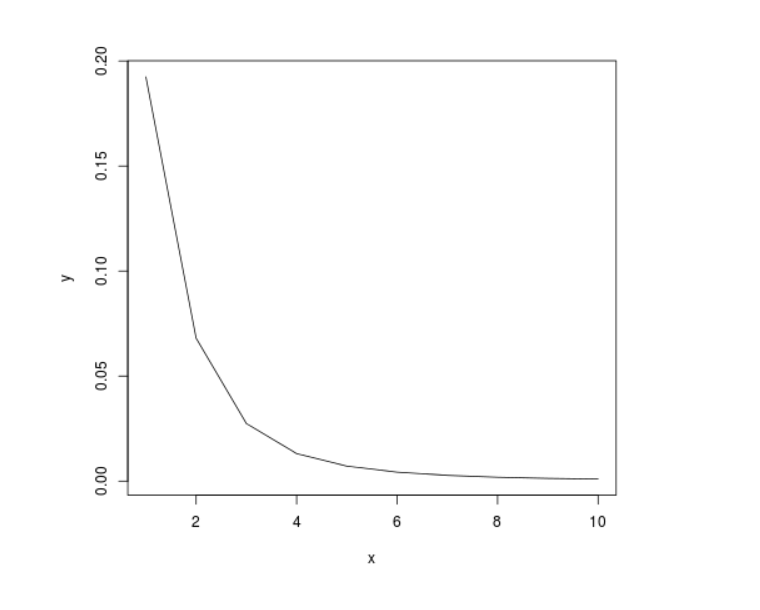# Perform Probability Density Analysis on t-Distribution in R Programming – dt() Function

`dt()` function in R Language is used to return the probability density analysis on the Student t-distribution with random variable x and degree of freedom df.

Syntax: dt(x, df)

Parameters:
x: Random Variable
df: Degree of Freedom

Example 1:

 `# R Program to perform ` `# Probability Density Analysis ` ` `  `# Calling dt() Function ` `dt(``0``, ``10``) ` `dt(``1``, ``15``) ` `dt(``3``, ``40``) `

Output:

``` 0.3891084
 0.2341248
 0.006185947
```

Example 2:

 `# R Program to perform ` `# Probability Density Analysis ` ` `  `# Creating a vector ` `x <``-` `seq(``1``, ``10``, by ``=` `1``) ` ` `  `# Calling dt() Function ` `y <``-` `dt(x, ``2``) ` ` `  `# Plotting probability distribution graph ` `plot(x, y, ``type` `=``"l"``) `

Output:Whether you're preparing for your first job interview or aiming to upskill in this ever-evolving tech landscape, GeeksforGeeks Courses are your key to success. We provide top-quality content at affordable prices, all geared towards accelerating your growth in a time-bound manner. Join the millions we've already empowered, and we're here to do the same for you. Don't miss out - check it out now!

Previous
Next